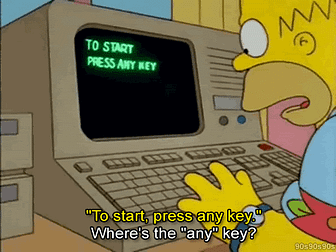Your guide for nailing the technical interviewLessons Learned: HashMaps
Question: Invert a binary tree. Input: 4 / \ 2 7 / \ / \ 1 3 6 9 Output: 4 / \ 7 2 / \ / \ 9 6 3 1 Solution Approach 1: Recursive The inverse of an…
Alice and Bob take turns playing a game, with Alice starting first. Initially, there is a number N on the chalkboard. On each player's turn, that player…
Question: Given a non-empty array of integers nums, every element appears twice except for one. Find that single one. Solution Approach 1: Hash Table We…Given an array A of integers, for each integer A[i] we may choose any x with -K <= x <= K, and add x to A[i]. After this process, we have some array B…Lessons Learned: TreeSet, Array ManipulationQuestion: Implement an algorithm to print all valid (properly opened and closed) combinations of n pairs of parentheses.
See all